CGAL 5.3 - Manual
GIS (Geographic Information System)

Many sensors used in GIS applications (for example LIDAR), generate dense point clouds. Such applications often take advantage of more advanced data structures: for example, Triangulated Irregular Networks (TIN) that can be the base for Digital Evelation Models (DEM) and in particular for the generation of Digital Terrain Models (DTM). Point clouds can also be enriched by classification information that segments the points into ground, vegetation and building points (or other user-defined labels).

The definitions of some data structures may vary according to different sources. We will use the following terms within this tutorial:

• TIN: Triangulated Irregular Network, a 2D triangulation structure that connects 3D points based on their projections on the horizontal plane.
• DSM: Digital Surface Model, a model of the whole scanned surface including buildings and vegetation. We use a TIN to store the DSM.
• DTM: Digital Terrain Model, a model of the bare ground surface without objects like buildings or vegetation. We both use a TIN and a raster to store a DTM.
• DEM: Digital Elevation Model, a more general term that includes both DSM and DTM.

This tutorial illustrates the following scenario. From an input point cloud, we first compute a DSM stored as a TIN. Then, we filter out overly large facets that correspond either to building facades or to vegetation noise. Large components corresponding to the ground are kept. Holes are filled and the obtained DEM is remeshed. A raster DEM and a set of contour polylines are generated from it. Finally, supervised 3-label classification is performed to segment vegetation, building and group points.

# Triangulated Irregular Network (TIN)

CGAL provides several triangulation data structures and algorithms. A TIN can be generated by combining the 2D Delaunay triangulation with projection traits: the triangulation structure is computed using the 2D positions of the points along a selected plane (usually, the XY-plane), while the 3D positions of the points are kept for visualization and measurements.

A TIN data structure can thus simply be defined the following way:

using Projection_traits = CGAL::Projection_traits_xy_3<Kernel>;
using Point_2 = Kernel::Point_2;
using Point_3 = Kernel::Point_3;
using Segment_3 = Kernel::Segment_3;
// Triangulated Irregular Network

# Digital Surface Model (DSM)

Point clouds in many formats (XYZ, OFF, PLY, LAS) can be easily loaded into a CGAL::Point_set_3 structure, using the stream operator. Generating a DSM stored in TIN is then straightforward:

std::ifstream ifile (argv, std::ios_base::binary);
ifile >> points;
std::cerr << points.size() << " point(s) read" << std::endl;
// Create DSM
TIN dsm (points.points().begin(), points.points().end());

Because the Delaunay triangulation of CGAL is a model of FaceGraph, it is straightforward to convert the generated TIN into a mesh structure such as CGAL::Surface_mesh and be saved into whatever formats are supported by such structure:

Mesh dsm_mesh;
CGAL::copy_face_graph (dsm, dsm_mesh);
std::ofstream dsm_ofile ("dsm.ply", std::ios_base::binary);
CGAL::IO::write_PLY (dsm_ofile, dsm_mesh);
dsm_ofile.close();

An example of a DSM computed this way on the San Fransisco data set (see References) is given in Figure 0.1.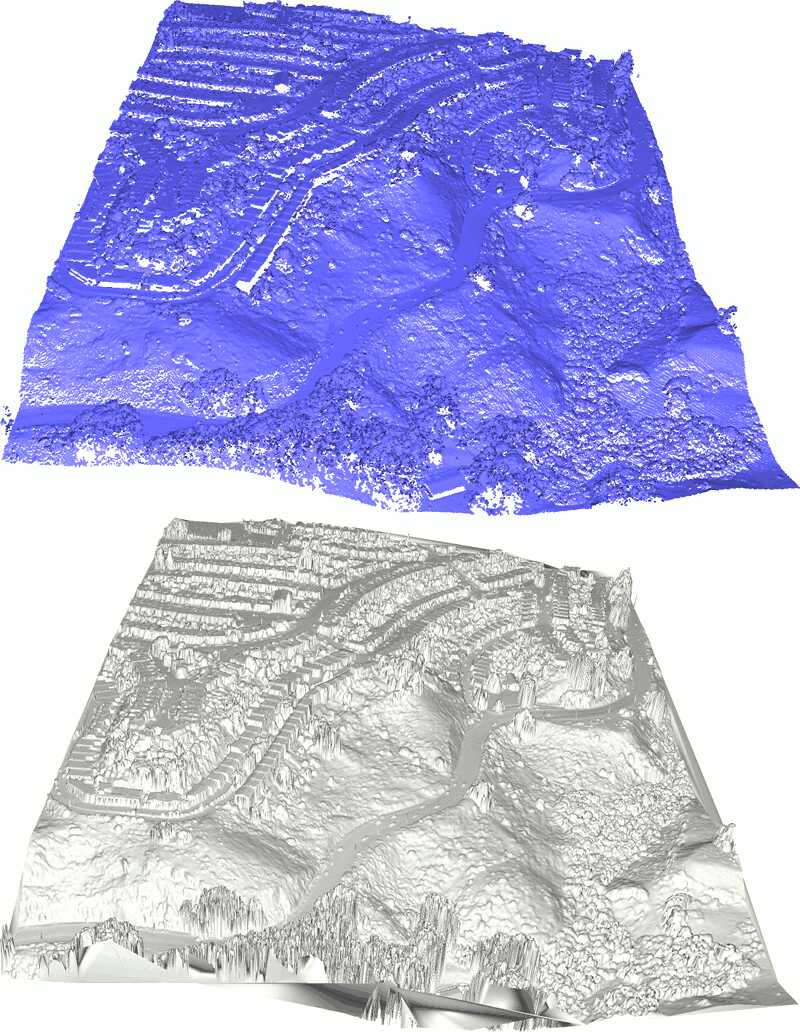Figure 0.1 Input point cloud and output DSM.

# Digital Terrain Model (DTM)

The generated DSM is used as a base for DTM computation, that is a representation of the ground as another TIN after filtering non-ground points.

We propose, as an example, a simple DTM estimation decomposed in the following steps:

1. Thresholding the height of the facets to remove brutal changes of elevation
2. Clustering the other facets into connected components
3. Filtering all components smaller than a user-defined threshold

This algorithm relies on 2 parameters: a height threshold that corresponds to the minimum height of a building, and a perimeter threshold that corresponds to the maximum size of a building on the 2D projection.

## TIN With Info

Because it takes advantage of the flexible CGAL Delaunay triangulation API, our TIN can be enriched with information on vertices and/or on faces. In our case, each vertex keeps track of the index of the corresponding point in the input point cloud (which will allow to filter ground points afterwards), and each face is given the index of its connected component.

auto idx_to_point_with_info
= [&](const Point_set::Index& idx) -> std::pair<Point_3, Point_set::Index>
{
return std::make_pair (points.point(idx), idx);
};
TIN_with_info tin_with_info
(boost::make_transform_iterator (points.begin(), idx_to_point_with_info),
boost::make_transform_iterator (points.end(), idx_to_point_with_info));

## Identifying Connected Components

Connected components are identified through a flooding algorithm: from a seed face, all incident faces are inserted in the current connected component unless their heights exceed the user-defined threshold.

double spacing = CGAL::compute_average_spacing<Concurrency_tag>(points, 6);
spacing *= 2;
auto face_height
= [&](const TIN_with_info::Face_handle fh) -> double
{
double out = 0.;
for (int i = 0; i < 3; ++ i)
out = (std::max) (out, CGAL::abs(fh->vertex(i)->point().z() - fh->vertex((i+1)%3)->point().z()));
return out;
};
// Initialize faces info
for (TIN_with_info::Face_handle fh : tin_with_info.all_face_handles())
if (tin_with_info.is_infinite(fh) || face_height(fh) > spacing) // Filtered faces are given info() = -2
fh->info() = -2;
else // Pending faces are given info() = -1;
fh->info() = -1;
// Flooding algorithm
std::vector<int> component_size;
for (TIN_with_info::Face_handle fh : tin_with_info.finite_face_handles())
{
if (fh->info() != -1)
continue;
std::queue<TIN_with_info::Face_handle> todo;
todo.push(fh);
int size = 0;
while (!todo.empty())
{
TIN_with_info::Face_handle current = todo.front();
todo.pop();
if (current->info() != -1)
continue;
current->info() = int(component_size.size());
++ size;
for (int i = 0; i < 3; ++ i)
todo.push (current->neighbor(i));
}
component_size.push_back (size);
}
std::cerr << component_size.size() << " connected component(s) found" << std::endl;

This TIN enriched with connected component information can be saved as a colored mesh:

Mesh tin_colored_mesh;
Mesh::Property_map<Mesh::Face_index, CGAL::IO::Color>
CGAL::copy_face_graph (tin_with_info, tin_colored_mesh,
CGAL::parameters::face_to_face_output_iterator
(boost::make_function_output_iterator
([&](const std::pair<TIN_with_info::Face_handle, Mesh::Face_index>& ff)
{
// Color unassigned faces gray
if (ff.first->info() < 0)
color_map[ff.second] = CGAL::IO::Color(128, 128, 128);
else
{
// Random color seeded by the component ID
CGAL::Random r (ff.first->info());
color_map[ff.second] = CGAL::IO::Color (r.get_int(64, 192),
r.get_int(64, 192),
r.get_int(64, 192));
}
})));
std::ofstream tin_colored_ofile ("colored_tin.ply", std::ios_base::binary);
CGAL::IO::set_binary_mode (tin_colored_ofile);
CGAL::IO::write_PLY (tin_colored_ofile, tin_colored_mesh);
tin_colored_ofile.close();

An example of a TIN colored by connected components is given in Figure 0.2.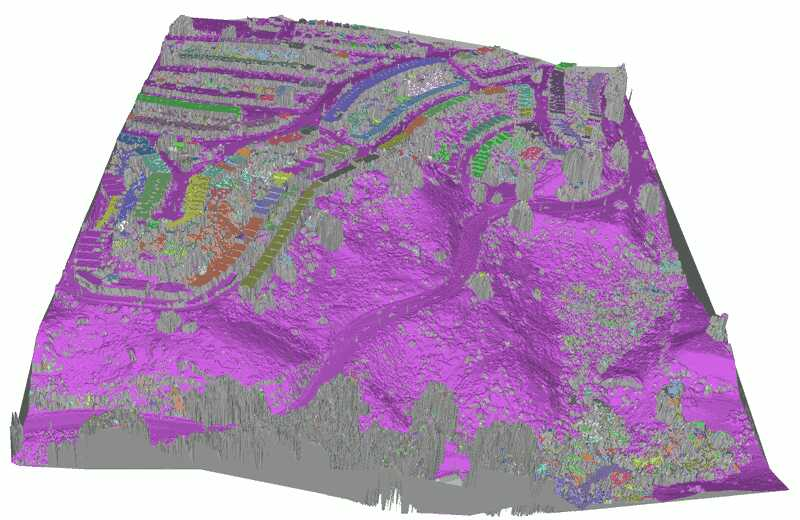Figure 0.2 TIN colored by connected components. Faces above height threshold are not assigned to any component and are displayed in gray.

## Filtering

Components smaller than the largest building can be removed this way:

int min_size = int(points.size() / 2);
std::vector<TIN_with_info::Vertex_handle> to_remove;
for (TIN_with_info::Vertex_handle vh : tin_with_info.finite_vertex_handles())
{
TIN_with_info::Face_circulator circ = tin_with_info.incident_faces (vh),
start = circ;
// Remove a vertex if it's only adjacent to components smaller than threshold
bool keep = false;
do
{
if (circ->info() >= 0 && component_size[std::size_t(circ->info())] > min_size)
{
keep = true;
break;
}
}
while (++ circ != start);
if (!keep)
to_remove.push_back (vh);
}
std::cerr << to_remove.size() << " vertices(s) will be removed after filtering" << std::endl;
for (TIN_with_info::Vertex_handle vh : to_remove)
tin_with_info.remove (vh);

## Hole Filling and Remeshing

Because simply removing vertices in the large areas covered by buildings results in large Delaunay faces that offer a poor 3D representation of the DTM, an additional step can help producing better shaped meshes: faces larger than a threshold are removed and filled with a hole filling algorithm that triangulates, refines and fairs the holes.

The following snippet copies the TIN into a mesh while filtering out overly large faces, then identifies the holes and fills them all except for the largest one (which is the outer hull).

// Copy and keep track of overly large faces
Mesh dtm_mesh;
std::vector<Mesh::Face_index> face_selection;
Mesh::Property_map<Mesh::Face_index, bool> face_selection_map
double limit = CGAL::square (5 * spacing);
CGAL::copy_face_graph (tin_with_info, dtm_mesh,
CGAL::parameters::face_to_face_output_iterator
(boost::make_function_output_iterator
([&](const std::pair<TIN_with_info::Face_handle, Mesh::Face_index>& ff)
{
double longest_edge = 0.;
bool border = false;
for (int i = 0; i < 3; ++ i)
{
longest_edge = (std::max)(longest_edge, CGAL::squared_distance
(ff.first->vertex((i+1)%3)->point(),
ff.first->vertex((i+2)%3)->point()));
TIN_with_info::Face_circulator circ
= tin_with_info.incident_faces (ff.first->vertex(i)),
start = circ;
do
{
if (tin_with_info.is_infinite (circ))
{
border = true;
break;
}
}
while (++ circ != start);
if (border)
break;
}
// Select if face is too big AND it's not
// on the border (to have closed holes)
if (!border && longest_edge > limit)
{
face_selection_map[ff.second] = true;
face_selection.push_back (ff.second);
}
})));
// Save original DTM
std::ofstream dtm_ofile ("dtm.ply", std::ios_base::binary);
CGAL::IO::write_PLY (dtm_ofile, dtm_mesh);
dtm_ofile.close();
std::cerr << face_selection.size() << " face(s) are selected for removal" << std::endl;
// Expand face selection to keep a well formed 2-manifold mesh after removal
CGAL::expand_face_selection_for_removal (face_selection, dtm_mesh, face_selection_map);
face_selection.clear();
for (Mesh::Face_index fi : faces(dtm_mesh))
if (face_selection_map[fi])
face_selection.push_back(fi);
std::cerr << face_selection.size() << " face(s) are selected for removal after expansion" << std::endl;
for (Mesh::Face_index fi : face_selection)
CGAL::Euler::remove_face (halfedge(fi, dtm_mesh), dtm_mesh);
dtm_mesh.collect_garbage();
if (!dtm_mesh.is_valid())
std::cerr << "Invalid mesh!" << std::endl;
// Save filtered DTM
std::ofstream dtm_holes_ofile ("dtm_with_holes.ply", std::ios_base::binary);
CGAL::IO::set_binary_mode (dtm_holes_ofile);
CGAL::IO::write_PLY (dtm_holes_ofile, dtm_mesh);
dtm_holes_ofile.close();
// Get all holes
std::vector<Mesh::Halfedge_index> holes;
CGAL::Polygon_mesh_processing::extract_boundary_cycles (dtm_mesh, std::back_inserter (holes));
std::cerr << holes.size() << " hole(s) identified" << std::endl;
// Identify outer hull (hole with maximum size)
double max_size = 0.;
Mesh::Halfedge_index outer_hull;
for (Mesh::Halfedge_index hi : holes)
{
CGAL::Bbox_3 hole_bbox;
for (Mesh::Halfedge_index haf : CGAL::halfedges_around_face(hi, dtm_mesh))
{
const Point_3& p = dtm_mesh.point(target(haf, dtm_mesh));
hole_bbox += p.bbox();
}
double size = CGAL::squared_distance (Point_2(hole_bbox.xmin(), hole_bbox.ymin()),
Point_2(hole_bbox.xmax(), hole_bbox.ymax()));
if (size > max_size)
{
max_size = size;
outer_hull = hi;
}
}
// Fill all holes except the bigest (which is the outer hull of the mesh)
for (Mesh::Halfedge_index hi : holes)
if (hi != outer_hull)
// Save DTM with holes filled
std::ofstream dtm_filled_ofile ("dtm_filled.ply", std::ios_base::binary);
CGAL::IO::set_binary_mode (dtm_filled_ofile);
CGAL::IO::write_PLY (dtm_filled_ofile, dtm_mesh);
dtm_filled_ofile.close();

Isotropic remeshing can also be performed as a final step in order to produce a more regular mesh that is not constrained by the shape of 2D Delaunay faces.

CGAL::Polygon_mesh_processing::isotropic_remeshing (faces(dtm_mesh), spacing, dtm_mesh);
std::ofstream dtm_remeshed_ofile ("dtm_remeshed.ply", std::ios_base::binary);
CGAL::IO::set_binary_mode (dtm_remeshed_ofile);
CGAL::IO::write_PLY (dtm_remeshed_ofile, dtm_mesh);
dtm_remeshed_ofile.close();

Figure 0.3 shows how these different steps affect the output mesh and Figure 0.4 shows the DTM isotropic mesh.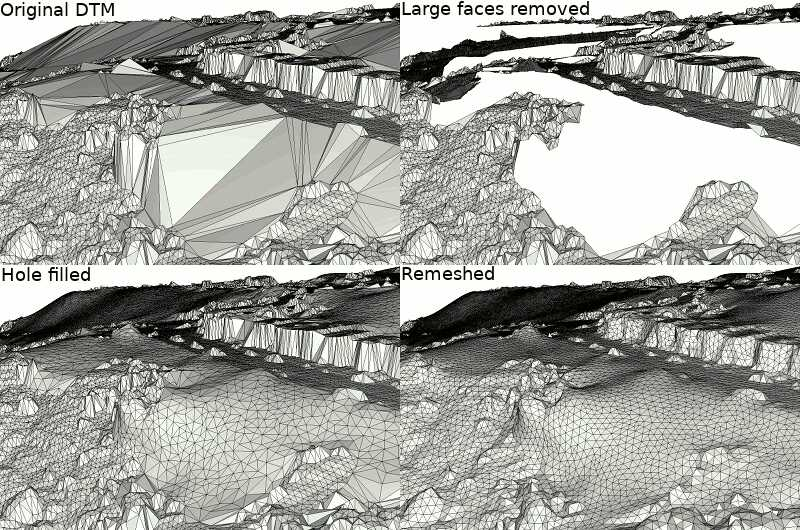Figure 0.3 Raw DTM and postprocessing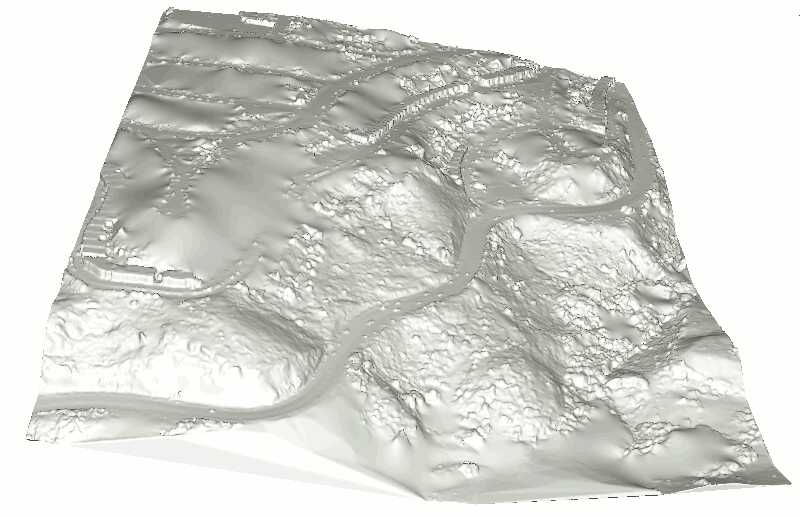Figure 0.4 Final DTM.

# Rastering

The TIN data structure can be combined with barycentric coordinates in order to interpolate and thus rasterize a height map at any resolution needed the information embedded in the vertices.

Because the latest two steps (hole filling and remeshing) were performed on a 3D mesh, the hypothesis that our DTM is a 2.5D representation may no longer be valid. We thus first rebuild a TIN using the vertices of the isotropic DTM mesh lastly computed.

The following snippet generates a raster image of the height in the form of rainbow ramp PPM file (simple bitmap format):

CGAL::Bbox_3 bbox = CGAL::bbox_3 (points.points().begin(), points.points().end());
// Generate raster image 1920-pixels large
std::size_t width = 1920;
std::size_t height = std::size_t((bbox.ymax() - bbox.ymin()) * 1920 / (bbox.xmax() - bbox.xmin()));
std::cerr << "Rastering with resolution " << width << "x" << height << std::endl;
// Use PPM format (Portable PixMap) for simplicity
std::ofstream raster_ofile ("raster.ppm", std::ios_base::binary);
raster_ofile << "P6" << std::endl // magic number
<< width << " " << height << std::endl // dimensions of the image
<< 255 << std::endl; // maximum color value
// Use rainbow color ramp output
Color_ramp color_ramp;
// Keeping track of location from one point to its neighbor allows
// for fast locate in DT
TIN::Face_handle location;
// Query each pixel of the image
for (std::size_t y = 0; y < height; ++ y)
for (std::size_t x = 0; x < width; ++ x)
{
Point_3 query (bbox.xmin() + x * (bbox.xmax() - bbox.xmin()) / double(width),
bbox.ymin() + (height-y) * (bbox.ymax() - bbox.ymin()) / double(height),
0); // not relevant for location in 2D
location = dtm_clean.locate (query, location);
// Points outside the convex hull will be colored black
std::array<unsigned char, 3> colors { 0, 0, 0 };
if (!dtm_clean.is_infinite(location))
{
std::array<double, 3> barycentric_coordinates
(Point_2 (location->vertex(0)->point().x(), location->vertex(0)->point().y()),
Point_2 (location->vertex(1)->point().x(), location->vertex(1)->point().y()),
Point_2 (location->vertex(2)->point().x(), location->vertex(2)->point().y()),
Point_2 (query.x(), query.y()),
Kernel());
double height_at_query
= (barycentric_coordinates * location->vertex(0)->point().z()
+ barycentric_coordinates * location->vertex(1)->point().z()
+ barycentric_coordinates * location->vertex(2)->point().z());
// Color ramp generates a color depending on a value from 0 to 1
double height_ratio = (height_at_query - bbox.zmin()) / (bbox.zmax() - bbox.zmin());
colors = color_ramp.get(height_ratio);
}
raster_ofile.write ((char*)(&colors), 3);
}
raster_ofile.close();

An example of a raster image with a rainbow ramp representing height is given in Figure 0.5.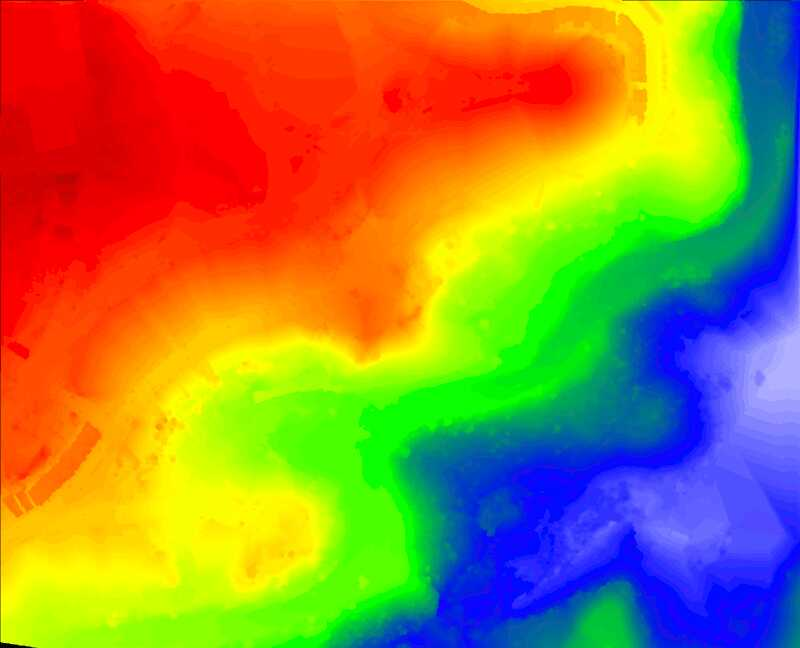Figure 0.5 Raster visualisation of height using a rainbow ramp, ranging from light blue for low values to dark red for high values.

# Contouring

Extracting isolevels of a function defined on a TIN is another application that can be done with CGAL. We demonstrate here how to extract isolevels of height to build a topographic map.

## Building a Contour Graph

The first step is to extract, from all faces of the triangulation, the section of each isolevel that goes through that face, in the form of a segment. The following functions allow to test if one isovalue does cross a face, and to extract it then:

bool face_has_isovalue (TIN::Face_handle fh, double isovalue)
{
bool above = false, below = false;
for (int i = 0; i < 3; ++ i)
{
// Face has isovalue if one of its vertices is above and another
// one below
if (fh->vertex(i)->point().z() > isovalue)
above = true;
if (fh->vertex(i)->point().z() < isovalue)
below = true;
}
return (above && below);
}
Segment_3 isocontour_in_face (TIN::Face_handle fh, double isovalue)
{
Point_3 source;
Point_3 target;
bool source_found = false;
for (int i = 0; i < 3; ++ i)
{
Point_3 p0 = fh->vertex((i+1) % 3)->point();
Point_3 p1 = fh->vertex((i+2) % 3)->point();
// Check if the isovalue crosses segment (p0,p1)
if ((p0.z() - isovalue) * (p1.z() - isovalue) > 0)
continue;
double zbottom = p0.z();
double ztop = p1.z();
if (zbottom > ztop)
{
std::swap (zbottom, ztop);
std::swap (p0, p1);
}
// Compute position of segment vertex
double ratio = (isovalue - zbottom) / (ztop - zbottom);
Point_3 p = CGAL::barycenter (p0, (1 - ratio), p1,ratio);
if (source_found)
target = p;
else
{
source = p;
source_found = true;
}
}
return Segment_3 (source, target);
}

From these functions, we can create a graph of segments to process later into a set of polylines. To do so, we use the boost::adjacency_list structure and keep track of a mapping from the positions of the end points to the vertices of the graph.

The following code computes 50 isovalues evenly distributed between the minimum and maximum heights of the point cloud and creates a graph containing all isolevels:

std::array<double, 50> isovalues; // Contour 50 isovalues
for (std::size_t i = 0; i < isovalues.size(); ++ i)
isovalues[i] = bbox.zmin() + ((i+1) * (bbox.zmax() - bbox.zmin()) / (isovalues.size() - 2));
// First find on each face if they are crossed by some isovalues and
// extract segments in a graph
using Segment_graph = boost::adjacency_list<boost::listS, boost::vecS, boost::undirectedS, Point_3>;
Segment_graph graph;
using Map_p2v = std::map<Point_3, Segment_graph::vertex_descriptor>;
Map_p2v map_p2v;
for (TIN::Face_handle vh : dtm_clean.finite_face_handles())
for (double iv : isovalues)
if (face_has_isovalue (vh, iv))
{
Segment_3 segment = isocontour_in_face (vh, iv);
for (const Point_3& p : { segment.source(), segment.target() })
{
// Only insert end points of segments once to get a well connected graph
Map_p2v::iterator iter;
bool inserted;
std::tie (iter, inserted) = map_p2v.insert (std::make_pair (p, Segment_graph::vertex_descriptor()));
if (inserted)
{
graph[iter->second] = p;
}
}
}

## Splitting Into Polylines

Once the graph is created, splitting it into polylines is easily performed using the function CGAL::split_graph_into_polylines() :

// Split segments into polylines
std::vector<std::vector<Point_3> > polylines;
Polylines_visitor<Segment_graph> visitor (graph, polylines);
std::cerr << polylines.size() << " polylines computed, with "
<< map_p2v.size() << " vertices in total" << std::endl;
// Output to WKT file
std::ofstream contour_ofile ("contour.wkt");
contour_ofile.precision(18);
CGAL::IO::write_multi_linestring_WKT (contour_ofile, polylines);
contour_ofile.close();

This function requires a visitor which is called when starting a polyline, when adding a point to it and when ending it. Defining such a class is straightforward in our case:

template <typename Graph>
class Polylines_visitor
{
private:
std::vector<std::vector<Point_3> >& polylines;
Graph& graph;
public:
Polylines_visitor (Graph& graph, std::vector<std::vector<Point_3> >& polylines)
: polylines (polylines), graph(graph) { }
void start_new_polyline()
{
polylines.push_back (std::vector<Point_3>());
}
{
polylines.back().push_back (graph[vd]);
}
void end_polyline()
{
// filter small polylines
if (polylines.back().size() < 50)
polylines.pop_back();
}
};

## Simplifying

Because the output is quite noisy, users may want to simplify the polylines. CGAL provides a polyline simplification algorithm that guarantees that two polylines won't intersect after simplification. This algorithm takes advantage of the CGAL::Constrained_triangulation_plus_2, which embeds polylines as a set of constraints:

using CDT_vertex_base = PS::Vertex_base_2<Projection_traits>;

The following code simplifies the polyline set based on the squared distance to the original polylines, stopping when no more vertex can be removed without going farther than 4 times the average spacing.

// Construct constrained Delaunay triangulation with polylines as constraints
CTP ctp;
for (const std::vector<Point_3>& poly : polylines)
ctp.insert_constraint (poly.begin(), poly.end());
// Simplification algorithm with limit on distance
PS::simplify (ctp, PS::Squared_distance_cost(), PS::Stop_above_cost_threshold (16 * spacing * spacing));
polylines.clear();
for (CTP::Constraint_id cid : ctp.constraints())
{
polylines.push_back (std::vector<Point_3>());
polylines.back().reserve (ctp.vertices_in_constraint (cid).size());
for (CTP::Vertex_handle vh : ctp.vertices_in_constraint(cid))
polylines.back().push_back (vh->point());
}
std::size_t nb_vertices
= std::accumulate (polylines.begin(), polylines.end(), 0u,
[](std::size_t size, const std::vector<Point_3>& poly) -> std::size_t
{ return size + poly.size(); });
std::cerr << nb_vertices
<< " vertices remaining after simplification ("
<< 100. * (nb_vertices / double(map_p2v.size())) << "%)" << std::endl;
// Output to WKT file
std::ofstream simplified_ofile ("simplified.wkt");
simplified_ofile.precision(18);
CGAL::IO::write_multi_linestring_WKT (simplified_ofile, polylines);
simplified_ofile.close();

Examples of contours and simplifications are given in Figure 0.6.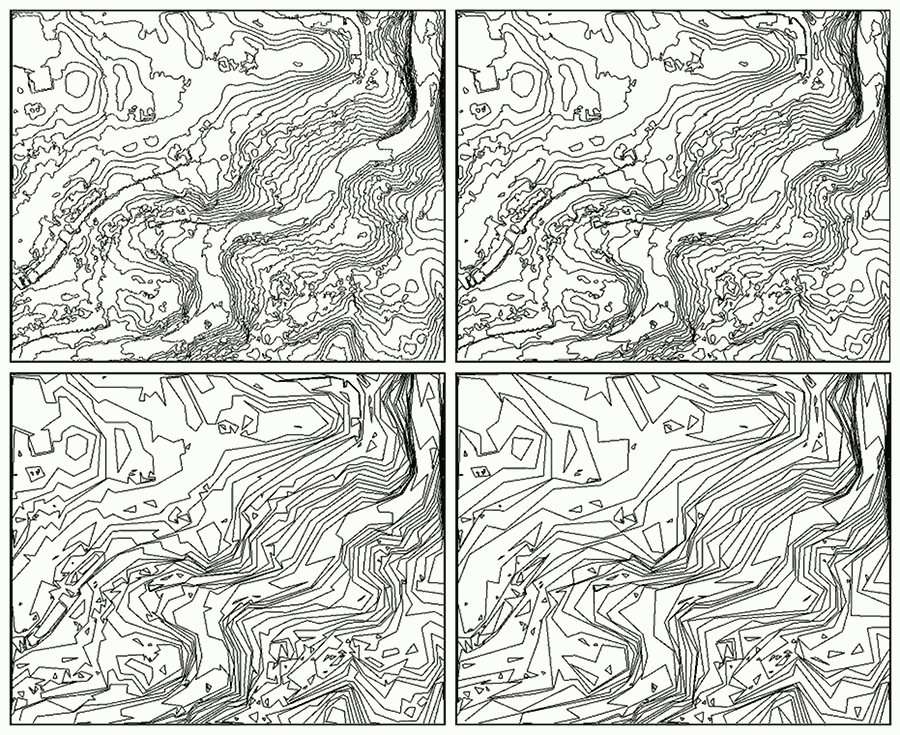Figure 0.6 Contouring using 50 isovalues evenly spaced. Top: original contouring using 148k vertices and simplification with a tolerance equal to the average spacing of the input point cloud (3.4% of the original polyline vertices remaining). Bottom: simplification with tolerance 4 times the average spacing (1.3% of vertices remaining) and with tolerance 10 times the average spacing (0.9% of vertices remaining). Polylines are intersection-free in all cases.

# Classifying

CGAL provides a package Classification which can be used to segment a point cloud into a user-defined label set. The state-of-the-art classifier currently available in CGAL is the random forest from ETHZ. As it is a supervised classifier, a training set is needed.

The following snippet shows how to use some manually selected training set to train a random forest classifier and compute a classification regularized by a graph cut algorithm:

// Get training from input
Point_set::Property_map<int> training_map;
bool training_found;
std::tie (training_map, training_found) = points.property_map<int>("training");
if (training_found)
{
std::cerr << "Classifying ground/vegetation/building" << std::endl;
// Create labels
Classification::Label_set labels ({ "ground", "vegetation", "building" });
// Generate features
Classification::Feature_set features;
Classification::Point_set_feature_generator<Kernel, Point_set, Point_set::Point_map>
generator (points, points.point_map(), 5); // 5 scales
// If TBB is used, features can be computed in parallel
generator.generate_point_based_features (features);
#else
generator.generate_point_based_features (features);
#endif
// Train a random forest classifier
Classification::ETHZ::Random_forest_classifier classifier (labels, features);
classifier.train (points.range(training_map));
// Classify with graphcut regularization
Classification::classify_with_graphcut<Concurrency_tag>
(points, points.point_map(), labels, classifier,
generator.neighborhood().k_neighbor_query(12), // regularize on 12-neighbors graph
0.5f, // graphcut weight
12, // Subdivide to speed-up process
label_map);
// Evaluate
std::cerr << "Mean IoU on training data = "
<< Classification::Evaluation(labels,
points.range(training_map),
points.range(label_map)).mean_intersection_over_union() << std::endl;
// Save the classified point set
std::ofstream classified_ofile ("classified.ply");
CGAL::IO::set_binary_mode (classified_ofile);
classified_ofile << points;
classified_ofile.close();
}

An example of training set and resulting classification is given in Figure 0.7.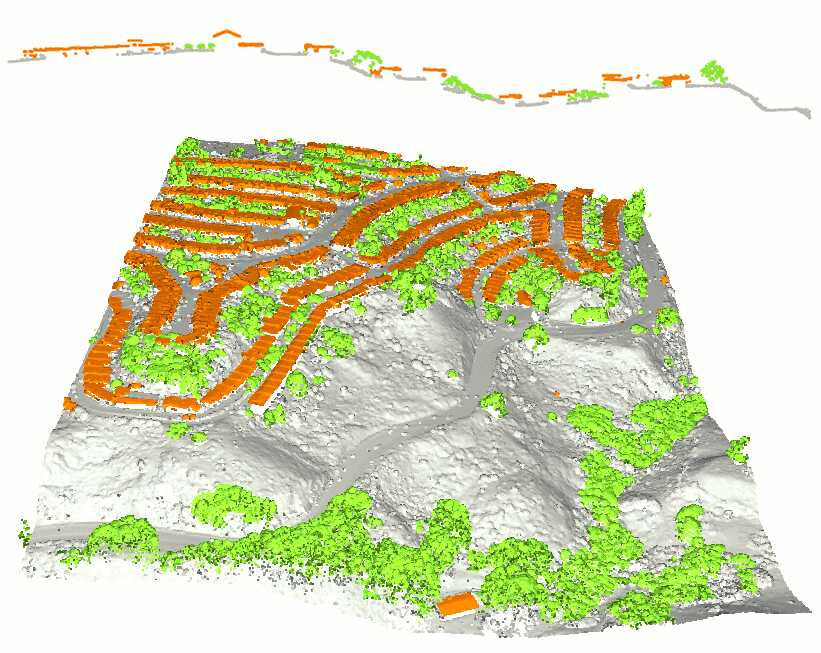Figure 0.7 Top: a slice of the point cloud classified by hand. Bottom: a classification regularized by graph cut after training on 3 manually classified slices.

# Full Code Example

All the code snippets used in this tutorial can be assembled to create a full GIS pipeline (provided the correct includes are used). We give a full code example which achieves all the steps described in this tutorial.

#include <CGAL/Exact_predicates_inexact_constructions_kernel.h>
#include <CGAL/Projection_traits_xy_3.h>
#include <CGAL/Delaunay_triangulation_2.h>
#include <CGAL/Triangulation_vertex_base_with_info_2.h>
#include <CGAL/Triangulation_face_base_with_info_2.h>
#include <CGAL/boost/graph/graph_traits_Delaunay_triangulation_2.h>
#include <CGAL/boost/graph/copy_face_graph.h>
#include <CGAL/Point_set_3.h>
#include <CGAL/Point_set_3/IO.h>
#include <CGAL/compute_average_spacing.h>
#include <CGAL/Surface_mesh.h>
#include <CGAL/Polygon_mesh_processing/locate.h>
#include <CGAL/Polygon_mesh_processing/triangulate_hole.h>
#include <CGAL/Polygon_mesh_processing/border.h>
#include <CGAL/Polygon_mesh_processing/remesh.h>
#include <CGAL/boost/graph/split_graph_into_polylines.h>
#include <CGAL/IO/WKT.h>
#include <CGAL/Constrained_Delaunay_triangulation_2.h>
#include <CGAL/Constrained_triangulation_plus_2.h>
#include <CGAL/Polyline_simplification_2/simplify.h>
#include <CGAL/Polyline_simplification_2/Squared_distance_cost.h>
#include <CGAL/Classification.h>
#include <CGAL/Random.h>
#include <fstream>
#include <queue>
#include "include/Color_ramp.h"
using Projection_traits = CGAL::Projection_traits_xy_3<Kernel>;
using Point_2 = Kernel::Point_2;
using Point_3 = Kernel::Point_3;
using Segment_3 = Kernel::Segment_3;
// Triangulated Irregular Network
// Triangulated Irregular Network (with info)
using Point_set = CGAL::Point_set_3<Point_3>;
namespace Classification = CGAL::Classification;
using Concurrency_tag = CGAL::Parallel_tag;
#else
using Concurrency_tag = CGAL::Sequential_tag;
#endif
bool face_has_isovalue (TIN::Face_handle fh, double isovalue)
{
bool above = false, below = false;
for (int i = 0; i < 3; ++ i)
{
// Face has isovalue if one of its vertices is above and another
// one below
if (fh->vertex(i)->point().z() > isovalue)
above = true;
if (fh->vertex(i)->point().z() < isovalue)
below = true;
}
return (above && below);
}
Segment_3 isocontour_in_face (TIN::Face_handle fh, double isovalue)
{
Point_3 source;
Point_3 target;
bool source_found = false;
for (int i = 0; i < 3; ++ i)
{
Point_3 p0 = fh->vertex((i+1) % 3)->point();
Point_3 p1 = fh->vertex((i+2) % 3)->point();
// Check if the isovalue crosses segment (p0,p1)
if ((p0.z() - isovalue) * (p1.z() - isovalue) > 0)
continue;
double zbottom = p0.z();
double ztop = p1.z();
if (zbottom > ztop)
{
std::swap (zbottom, ztop);
std::swap (p0, p1);
}
// Compute position of segment vertex
double ratio = (isovalue - zbottom) / (ztop - zbottom);
Point_3 p = CGAL::barycenter (p0, (1 - ratio), p1,ratio);
if (source_found)
target = p;
else
{
source = p;
source_found = true;
}
}
return Segment_3 (source, target);
}
template <typename Graph>
class Polylines_visitor
{
private:
std::vector<std::vector<Point_3> >& polylines;
Graph& graph;
public:
Polylines_visitor (Graph& graph, std::vector<std::vector<Point_3> >& polylines)
: polylines (polylines), graph(graph) { }
void start_new_polyline()
{
polylines.push_back (std::vector<Point_3>());
}
{
polylines.back().push_back (graph[vd]);
}
void end_polyline()
{
// filter small polylines
if (polylines.back().size() < 50)
polylines.pop_back();
}
};
using CDT_vertex_base = PS::Vertex_base_2<Projection_traits>;
int main (int argc, char** argv)
{
if (argc != 2)
{
std::cerr << "Usage: " << argv << " points.ply" << std::endl;
return EXIT_FAILURE;
}
std::ifstream ifile (argv, std::ios_base::binary);
ifile >> points;
std::cerr << points.size() << " point(s) read" << std::endl;
// Create DSM
TIN dsm (points.points().begin(), points.points().end());
Mesh dsm_mesh;
CGAL::copy_face_graph (dsm, dsm_mesh);
std::ofstream dsm_ofile ("dsm.ply", std::ios_base::binary);
CGAL::IO::write_PLY (dsm_ofile, dsm_mesh);
dsm_ofile.close();
auto idx_to_point_with_info
= [&](const Point_set::Index& idx) -> std::pair<Point_3, Point_set::Index>
{
return std::make_pair (points.point(idx), idx);
};
TIN_with_info tin_with_info
(boost::make_transform_iterator (points.begin(), idx_to_point_with_info),
boost::make_transform_iterator (points.end(), idx_to_point_with_info));
double spacing = CGAL::compute_average_spacing<Concurrency_tag>(points, 6);
spacing *= 2;
auto face_height
= [&](const TIN_with_info::Face_handle fh) -> double
{
double out = 0.;
for (int i = 0; i < 3; ++ i)
out = (std::max) (out, CGAL::abs(fh->vertex(i)->point().z() - fh->vertex((i+1)%3)->point().z()));
return out;
};
// Initialize faces info
for (TIN_with_info::Face_handle fh : tin_with_info.all_face_handles())
if (tin_with_info.is_infinite(fh) || face_height(fh) > spacing) // Filtered faces are given info() = -2
fh->info() = -2;
else // Pending faces are given info() = -1;
fh->info() = -1;
// Flooding algorithm
std::vector<int> component_size;
for (TIN_with_info::Face_handle fh : tin_with_info.finite_face_handles())
{
if (fh->info() != -1)
continue;
std::queue<TIN_with_info::Face_handle> todo;
todo.push(fh);
int size = 0;
while (!todo.empty())
{
TIN_with_info::Face_handle current = todo.front();
todo.pop();
if (current->info() != -1)
continue;
current->info() = int(component_size.size());
++ size;
for (int i = 0; i < 3; ++ i)
todo.push (current->neighbor(i));
}
component_size.push_back (size);
}
std::cerr << component_size.size() << " connected component(s) found" << std::endl;
Mesh tin_colored_mesh;
Mesh::Property_map<Mesh::Face_index, CGAL::IO::Color>
CGAL::copy_face_graph (tin_with_info, tin_colored_mesh,
CGAL::parameters::face_to_face_output_iterator
(boost::make_function_output_iterator
([&](const std::pair<TIN_with_info::Face_handle, Mesh::Face_index>& ff)
{
// Color unassigned faces gray
if (ff.first->info() < 0)
color_map[ff.second] = CGAL::IO::Color(128, 128, 128);
else
{
// Random color seeded by the component ID
CGAL::Random r (ff.first->info());
color_map[ff.second] = CGAL::IO::Color (r.get_int(64, 192),
r.get_int(64, 192),
r.get_int(64, 192));
}
})));
std::ofstream tin_colored_ofile ("colored_tin.ply", std::ios_base::binary);
CGAL::IO::set_binary_mode (tin_colored_ofile);
CGAL::IO::write_PLY (tin_colored_ofile, tin_colored_mesh);
tin_colored_ofile.close();
int min_size = int(points.size() / 2);
std::vector<TIN_with_info::Vertex_handle> to_remove;
for (TIN_with_info::Vertex_handle vh : tin_with_info.finite_vertex_handles())
{
TIN_with_info::Face_circulator circ = tin_with_info.incident_faces (vh),
start = circ;
// Remove a vertex if it's only adjacent to components smaller than threshold
bool keep = false;
do
{
if (circ->info() >= 0 && component_size[std::size_t(circ->info())] > min_size)
{
keep = true;
break;
}
}
while (++ circ != start);
if (!keep)
to_remove.push_back (vh);
}
std::cerr << to_remove.size() << " vertices(s) will be removed after filtering" << std::endl;
for (TIN_with_info::Vertex_handle vh : to_remove)
tin_with_info.remove (vh);
// Copy and keep track of overly large faces
Mesh dtm_mesh;
std::vector<Mesh::Face_index> face_selection;
Mesh::Property_map<Mesh::Face_index, bool> face_selection_map
double limit = CGAL::square (5 * spacing);
CGAL::copy_face_graph (tin_with_info, dtm_mesh,
CGAL::parameters::face_to_face_output_iterator
(boost::make_function_output_iterator
([&](const std::pair<TIN_with_info::Face_handle, Mesh::Face_index>& ff)
{
double longest_edge = 0.;
bool border = false;
for (int i = 0; i < 3; ++ i)
{
longest_edge = (std::max)(longest_edge, CGAL::squared_distance
(ff.first->vertex((i+1)%3)->point(),
ff.first->vertex((i+2)%3)->point()));
TIN_with_info::Face_circulator circ
= tin_with_info.incident_faces (ff.first->vertex(i)),
start = circ;
do
{
if (tin_with_info.is_infinite (circ))
{
border = true;
break;
}
}
while (++ circ != start);
if (border)
break;
}
// Select if face is too big AND it's not
// on the border (to have closed holes)
if (!border && longest_edge > limit)
{
face_selection_map[ff.second] = true;
face_selection.push_back (ff.second);
}
})));
// Save original DTM
std::ofstream dtm_ofile ("dtm.ply", std::ios_base::binary);
CGAL::IO::write_PLY (dtm_ofile, dtm_mesh);
dtm_ofile.close();
std::cerr << face_selection.size() << " face(s) are selected for removal" << std::endl;
// Expand face selection to keep a well formed 2-manifold mesh after removal
CGAL::expand_face_selection_for_removal (face_selection, dtm_mesh, face_selection_map);
face_selection.clear();
for (Mesh::Face_index fi : faces(dtm_mesh))
if (face_selection_map[fi])
face_selection.push_back(fi);
std::cerr << face_selection.size() << " face(s) are selected for removal after expansion" << std::endl;
for (Mesh::Face_index fi : face_selection)
CGAL::Euler::remove_face (halfedge(fi, dtm_mesh), dtm_mesh);
dtm_mesh.collect_garbage();
if (!dtm_mesh.is_valid())
std::cerr << "Invalid mesh!" << std::endl;
// Save filtered DTM
std::ofstream dtm_holes_ofile ("dtm_with_holes.ply", std::ios_base::binary);
CGAL::IO::set_binary_mode (dtm_holes_ofile);
CGAL::IO::write_PLY (dtm_holes_ofile, dtm_mesh);
dtm_holes_ofile.close();
// Get all holes
std::vector<Mesh::Halfedge_index> holes;
CGAL::Polygon_mesh_processing::extract_boundary_cycles (dtm_mesh, std::back_inserter (holes));
std::cerr << holes.size() << " hole(s) identified" << std::endl;
// Identify outer hull (hole with maximum size)
double max_size = 0.;
Mesh::Halfedge_index outer_hull;
for (Mesh::Halfedge_index hi : holes)
{
CGAL::Bbox_3 hole_bbox;
for (Mesh::Halfedge_index haf : CGAL::halfedges_around_face(hi, dtm_mesh))
{
const Point_3& p = dtm_mesh.point(target(haf, dtm_mesh));
hole_bbox += p.bbox();
}
double size = CGAL::squared_distance (Point_2(hole_bbox.xmin(), hole_bbox.ymin()),
Point_2(hole_bbox.xmax(), hole_bbox.ymax()));
if (size > max_size)
{
max_size = size;
outer_hull = hi;
}
}
// Fill all holes except the bigest (which is the outer hull of the mesh)
for (Mesh::Halfedge_index hi : holes)
if (hi != outer_hull)
// Save DTM with holes filled
std::ofstream dtm_filled_ofile ("dtm_filled.ply", std::ios_base::binary);
CGAL::IO::set_binary_mode (dtm_filled_ofile);
CGAL::IO::write_PLY (dtm_filled_ofile, dtm_mesh);
dtm_filled_ofile.close();
CGAL::Polygon_mesh_processing::isotropic_remeshing (faces(dtm_mesh), spacing, dtm_mesh);
std::ofstream dtm_remeshed_ofile ("dtm_remeshed.ply", std::ios_base::binary);
CGAL::IO::set_binary_mode (dtm_remeshed_ofile);
CGAL::IO::write_PLY (dtm_remeshed_ofile, dtm_mesh);
dtm_remeshed_ofile.close();
TIN dtm_clean (dtm_mesh.points().begin(), dtm_mesh.points().end());
CGAL::Bbox_3 bbox = CGAL::bbox_3 (points.points().begin(), points.points().end());
// Generate raster image 1920-pixels large
std::size_t width = 1920;
std::size_t height = std::size_t((bbox.ymax() - bbox.ymin()) * 1920 / (bbox.xmax() - bbox.xmin()));
std::cerr << "Rastering with resolution " << width << "x" << height << std::endl;
// Use PPM format (Portable PixMap) for simplicity
std::ofstream raster_ofile ("raster.ppm", std::ios_base::binary);
raster_ofile << "P6" << std::endl // magic number
<< width << " " << height << std::endl // dimensions of the image
<< 255 << std::endl; // maximum color value
// Use rainbow color ramp output
Color_ramp color_ramp;
// Keeping track of location from one point to its neighbor allows
// for fast locate in DT
TIN::Face_handle location;
// Query each pixel of the image
for (std::size_t y = 0; y < height; ++ y)
for (std::size_t x = 0; x < width; ++ x)
{
Point_3 query (bbox.xmin() + x * (bbox.xmax() - bbox.xmin()) / double(width),
bbox.ymin() + (height-y) * (bbox.ymax() - bbox.ymin()) / double(height),
0); // not relevant for location in 2D
location = dtm_clean.locate (query, location);
// Points outside the convex hull will be colored black
std::array<unsigned char, 3> colors { 0, 0, 0 };
if (!dtm_clean.is_infinite(location))
{
std::array<double, 3> barycentric_coordinates
(Point_2 (location->vertex(0)->point().x(), location->vertex(0)->point().y()),
Point_2 (location->vertex(1)->point().x(), location->vertex(1)->point().y()),
Point_2 (location->vertex(2)->point().x(), location->vertex(2)->point().y()),
Point_2 (query.x(), query.y()),
Kernel());
double height_at_query
= (barycentric_coordinates * location->vertex(0)->point().z()
+ barycentric_coordinates * location->vertex(1)->point().z()
+ barycentric_coordinates * location->vertex(2)->point().z());
// Color ramp generates a color depending on a value from 0 to 1
double height_ratio = (height_at_query - bbox.zmin()) / (bbox.zmax() - bbox.zmin());
colors = color_ramp.get(height_ratio);
}
raster_ofile.write ((char*)(&colors), 3);
}
raster_ofile.close();
// Smooth heights with 5 successive Gaussian filters
double gaussian_variance = 4 * spacing * spacing;
for (TIN::Vertex_handle vh : dtm_clean.finite_vertex_handles())
{
double z = vh->point().z();
double total_weight = 1;
TIN::Vertex_circulator circ = dtm_clean.incident_vertices (vh),
start = circ;
do
{
if (!dtm_clean.is_infinite(circ))
{
double sq_dist = CGAL::squared_distance (vh->point(), circ->point());
double weight = std::exp(- sq_dist / gaussian_variance);
z += weight * circ->point().z();
total_weight += weight;
}
}
while (++ circ != start);
z /= total_weight;
vh->point() = Point_3 (vh->point().x(), vh->point().y(), z);
}
std::array<double, 50> isovalues; // Contour 50 isovalues
for (std::size_t i = 0; i < isovalues.size(); ++ i)
isovalues[i] = bbox.zmin() + ((i+1) * (bbox.zmax() - bbox.zmin()) / (isovalues.size() - 2));
// First find on each face if they are crossed by some isovalues and
// extract segments in a graph
using Segment_graph = boost::adjacency_list<boost::listS, boost::vecS, boost::undirectedS, Point_3>;
Segment_graph graph;
using Map_p2v = std::map<Point_3, Segment_graph::vertex_descriptor>;
Map_p2v map_p2v;
for (TIN::Face_handle vh : dtm_clean.finite_face_handles())
for (double iv : isovalues)
if (face_has_isovalue (vh, iv))
{
Segment_3 segment = isocontour_in_face (vh, iv);
for (const Point_3& p : { segment.source(), segment.target() })
{
// Only insert end points of segments once to get a well connected graph
Map_p2v::iterator iter;
bool inserted;
std::tie (iter, inserted) = map_p2v.insert (std::make_pair (p, Segment_graph::vertex_descriptor()));
if (inserted)
{
graph[iter->second] = p;
}
}
}
// Split segments into polylines
std::vector<std::vector<Point_3> > polylines;
Polylines_visitor<Segment_graph> visitor (graph, polylines);
std::cerr << polylines.size() << " polylines computed, with "
<< map_p2v.size() << " vertices in total" << std::endl;
// Output to WKT file
std::ofstream contour_ofile ("contour.wkt");
contour_ofile.precision(18);
CGAL::IO::write_multi_linestring_WKT (contour_ofile, polylines);
contour_ofile.close();
// Construct constrained Delaunay triangulation with polylines as constraints
CTP ctp;
for (const std::vector<Point_3>& poly : polylines)
ctp.insert_constraint (poly.begin(), poly.end());
// Simplification algorithm with limit on distance
PS::simplify (ctp, PS::Squared_distance_cost(), PS::Stop_above_cost_threshold (16 * spacing * spacing));
polylines.clear();
for (CTP::Constraint_id cid : ctp.constraints())
{
polylines.push_back (std::vector<Point_3>());
polylines.back().reserve (ctp.vertices_in_constraint (cid).size());
for (CTP::Vertex_handle vh : ctp.vertices_in_constraint(cid))
polylines.back().push_back (vh->point());
}
std::size_t nb_vertices
= std::accumulate (polylines.begin(), polylines.end(), 0u,
[](std::size_t size, const std::vector<Point_3>& poly) -> std::size_t
{ return size + poly.size(); });
std::cerr << nb_vertices
<< " vertices remaining after simplification ("
<< 100. * (nb_vertices / double(map_p2v.size())) << "%)" << std::endl;
// Output to WKT file
std::ofstream simplified_ofile ("simplified.wkt");
simplified_ofile.precision(18);
CGAL::IO::write_multi_linestring_WKT (simplified_ofile, polylines);
simplified_ofile.close();
// Get training from input
Point_set::Property_map<int> training_map;
bool training_found;
std::tie (training_map, training_found) = points.property_map<int>("training");
if (training_found)
{
std::cerr << "Classifying ground/vegetation/building" << std::endl;
// Create labels
Classification::Label_set labels ({ "ground", "vegetation", "building" });
// Generate features
Classification::Feature_set features;
Classification::Point_set_feature_generator<Kernel, Point_set, Point_set::Point_map>
generator (points, points.point_map(), 5); // 5 scales
// If TBB is used, features can be computed in parallel
generator.generate_point_based_features (features);
#else
generator.generate_point_based_features (features);
#endif
// Train a random forest classifier
Classification::ETHZ::Random_forest_classifier classifier (labels, features);
classifier.train (points.range(training_map));
// Classify with graphcut regularization
Classification::classify_with_graphcut<Concurrency_tag>
(points, points.point_map(), labels, classifier,
generator.neighborhood().k_neighbor_query(12), // regularize on 12-neighbors graph
0.5f, // graphcut weight
12, // Subdivide to speed-up process
label_map);
// Evaluate
std::cerr << "Mean IoU on training data = "
<< Classification::Evaluation(labels,
points.range(training_map),
points.range(label_map)).mean_intersection_over_union() << std::endl;
// Save the classified point set
std::ofstream classified_ofile ("classified.ply");
CGAL::IO::set_binary_mode (classified_ofile);
classified_ofile << points;
classified_ofile.close();
}
return EXIT_SUCCESS;
}

# References

This tutorial is based on the following CGAL packages:

The data set used throughout this tutorial comes from the https://www.usgs.gov/ database, licensed under the US Government Public Domain.